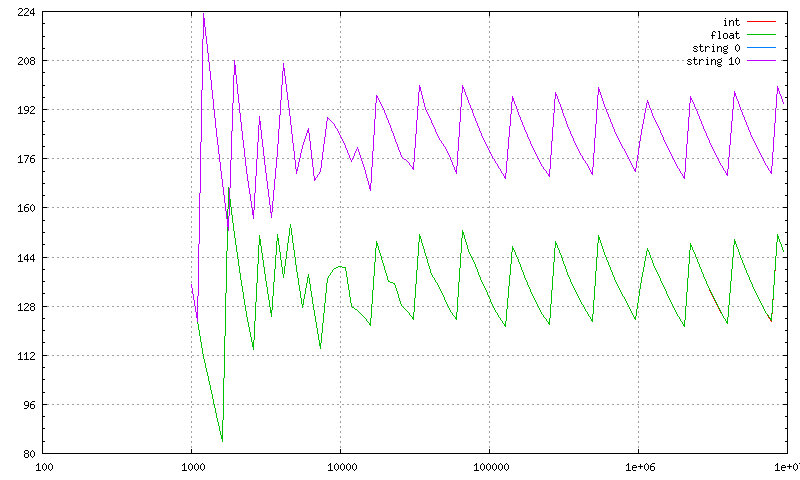Some observations about perl memory consumption

Perl is a rather big memory hog. These graphs show the memory consumption per element for arrays and hashes of various sizes containing various kinds of data: Integers, floating point numbers and (short) strings. The keys of the hash are just the (stringified) numbers from 0 to the number of elements minus 1.

All graphs were made on Linux systems. The total virtual memory (first field in /proc/\$\$/statm) is retrieved before and after populating the hash or array, and then divided by the number of elements. So it includes the actual data, overhead by perl data structures (SV, etc.), overhead by the malloc library, etc.For large arrays, the size per integer fluctuates between 20 and 28 bytes (which corresponds to 16 bytes for an IV + 4 bytes per array entry + plus possibly some wasted space because the array is extended exponentially). Storing FP values adds 8 bytes of memory, string values add another 16 bytes, so that even an empty string needs between 44 and 52 bytes. But note that in the space needed for an empty string you can also store strings up to 15 bytes (at least on this implementation which uses glibc's malloc), so the so the "string 0" line is hidden behind the "string 10" line. A 100 byte string (not shown here) needs about 100 bytes more, as expected.The situation is similar for hashes, except that they need more memory and fluctuate a lot more: An integer needs between 68 and 92 bytes, a 0-length string between 92 and 116 bytes. The size starts to rise again after more than ca. 1 million elements, probably because of the rising average key length.For arrays on a 64 bit system, there is no difference between integers and floating point numbers. Both need between 32 and 48 bytes. Strings need now between 80 and 96 bytes.Again we get two nice parallel sawtooth lines, fluctuating between 124 and 152 (integer or float) and 172 and 200 (string).

So depending on the type of data and the system you are on, a single (short) element in a hash or array may consume between 20 and 200 bytes. Roughly speaking there are three factors of two involved:

• Strings need twice as much memory as integers.
• Hashes need twice as much memory as arrays.
• A 64 bit system needs twice as much memory as a 32 bit system.